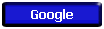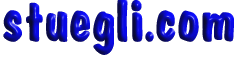# MORE ON ATOMS...

The atom above, made up of one proton and one electron, is called hydrogen (the abbreviation for hydrogen is H).  The proton and electron stay together because just like two magnets, the opposite electrical charges attract each other.  What keeps the two from crashing into each other?  The particles in an atom are not still.  The electron is constantly spinning around the center of the atom (called the nucleus).  The centrigugal force of the spinning electron keeps the two particles from coming into contact with each other much as the earth's rotation keeps it from plunging into the sun.

Keep in mind that atoms are extremely small.  One hydrogen atom, for example, is approximately 5 x 10-8 mm in diameter.  To put that in perspective, this dash - is approximately 1 mm in length, therefore it would take almost 20 million hydrogen atoms to make a line as long as the dash.  In the sub-atomic world, things often behave a bit strangely.  First of all, the electron actually spins very far from the nucleus.  If we were to draw the hydrogen atom above to scale, so that the proton were the size depicted above, the electron would actually be spinning approximately 0.5 km (or about a quarter of a mile) away from the nucleus.  In other words, if the proton was the size depicted above, the whole atom would be about the size of Giants Stadium.  Another peculiarity of this tiny world is the particles themselves.  Protons and neutrons behave like small particles, sort of like tiny billiard balls.  The electron however, has some of the properties of a wave.  In other words, the electron is more similar to a beam of light than it is to a billiard ball.  Thus to represent it as a small particle spinning around a nucleus is slightly misleading.  In actuality, the electron is a wave that surrounds the nucleus of an atom like a cloud.

While you should keep in mind that electrons actually form clouds around their nucleii, we will continue to represent the electron as a spinning particle to keep things simple.
In an electrically neutral atom, the positively charged protons are always balanced by an equal number of negatively charged electrons.  As we have seen, hydrogen is the simplest atom with only one proton and one electron.  Helium is the 2nd simplest atom.  It has two protons in its nucleus and two electrons spinning around the nucleus.  With helium though, we have to introduce another particle.  Because the 2 protons in the nucleus have the same charge on them, they would tend to repel each other, and the nucleus would fall apart.  To keep the nucleus from pushing apart, helium has two neutrons in its nucleus.  Neutrons have no electrical charge on them and act as a sort of nuclear glue, holding the protons, and thus the nucleus, together.

As you can see, helium is larger than hydrogen.  As you add electrons, protons and neutrons, the size of the atom increases.  We can measure an atom's size in two ways: using the atomic number (Z) or using the atomic mass (A, also known as the mass number).  The atomic number describes the number of protons in an atom.  For hydrogen the atomic number, Z, is equal to 1.  For helium Z = 2.  Since the number of protons equals the number of electrons in the neutral atom, Z also tells you the number of electrons in the atom.  The atomic mass tells you the number of protons plus neutrons in an atom.  Therefore, the atomic mass, A, of hydrogen is 1.  For helium A = 4.

Ions and Isotopes
So far we have only talked about electrically neutral atoms, atoms with no positive or negative charge on them.  Atoms, however, can have electrical charges.  Some atoms can either gain or lose electrons (the number of protons never changes in an atom).  If an atom gains electrons, the atom becomes negatively charged.  If the atom loses electrons, the atom becomes positively charged (because the number of positively charged protons will exceed the number of electrons).  An atom that carries an electrical charge is called an ion.  Listed below are three forms of hydrogen; 2 ions and the electrically neutral form.
Neither the number of protons nor neutrons changes in any of these ions, therefore both the atomic number and the atomic mass remain the same.  While the number of protons for a given atom never changes, the number of neutrons can change.  Two atoms with different numbers of neutrons are called isotopes.  For example, an isotope of hydrogen exists in which the atom contains 1 neutron (commonly called deuterium).  Since the atomic mass is the number of protons plus neutrons, two isotopes of an element will have different atomic masses (however the atomic number, Z, will remain the same).Copyright © 2005 -  S. B. EglI